Disclosure: This post may contain affiliate links, meaning when you click the links and make a purchase, we receive a commission.

# Excel Formula to Add Percentage Markup [with Calculator]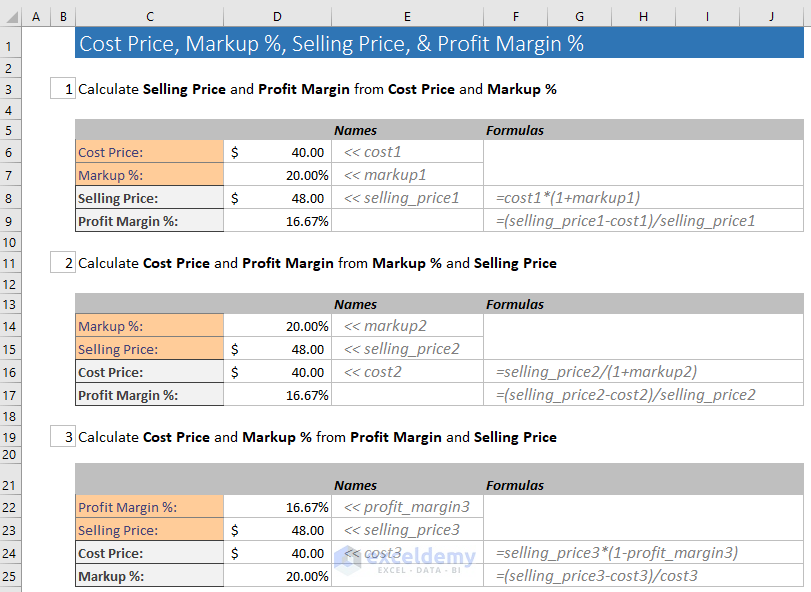In this tutorial, I will show several ways of using the Excel formula to add percentage markup to the cost price of a product. Adding markup % to the cost price will give you the selling price of the product.

## What is Markup?

Markup is actually the difference between the Selling Price and Wholesale or Making Cost of a product.

Again, we also calculate the Profit Margin in the same way. I mean Gross Profit Margin is also the difference between the Selling Price and Wholesale or Making Cost of the product.

But we calculate Markup % and Profit Margin % in different ways.

Here is the overall picture of these two concepts.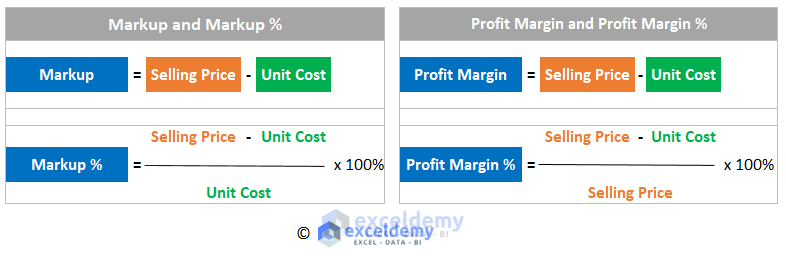You see that to get the Markup %, we divide the Profit Margin (= Selling Price – Unit Cost) by the Cost Price. And to calculate the Profit Margin %, we divide the Profit Margin (= Selling Price – Unit Cost) by the Selling Price.

## Adding Percentage Markup to the Cost Price (Example)

For example, your wholesale price (Cost Price) of a product is \$25. Now you want to add 40% Markup to the wholesale price of the product. What will be your selling price?

= Wholesale Price x (1+Markup %)

= \$25 x (1 + 40%)

= \$25 x 1.40

= \$35

Now, what is your Profit Margin and Profit Margin %?

Profit Margin = Selling Price – Unit Cost = \$35 – \$25 = \$10

Profit Margin % = Profit Margin / Selling Price = \$10 / \$35 =0.2857 = 0.2857 x 100% = 28.57%

## Excel Formula to Add Percentage Markup to a List of Products

Suppose, you have a list of products and you want to add different Markup % to those products. On one occasion, you can offer your customers one Markup % (say 30%) and on another occasion, you may offer a different Markup % (say 40%). All these pricing can be done in one Excel sheet.

You are seeing an Excel worksheet in the following image. A list of gardening related tools. Every product has a Wholesale Cost. We have calculated the Selling Prices of these products for different Markup Percentages (30%, 40%, 50%, 60%).

We have also calculated the Profit Margin for those Selling Prices.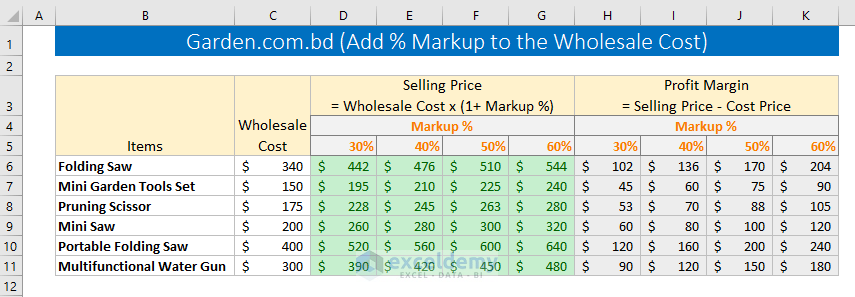### Excel formulas used to get the results

We have made just two Excel formulas to get the results.

1) In cell D6, I have used this Excel formula: =\$C6*(1+D\$5).

You see that this formula has mixed cell references. Column C and Row 5 is made absolute references.

We know when we go down or up, row references change. When we go left or right, column references change.

For the above formula, when we will go right, \$C will not change and when we shall copy the formula down, the row reference \$5 will not change.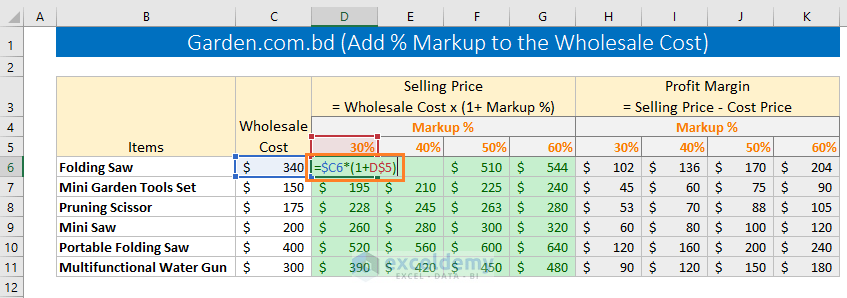2) In the cell H6, I have entered this formula: =D6-\$C6

This formula has one mixed reference: \$C6. As the column C is absolute, when we shall apply the formula on the right cells, the \$C reference will not change.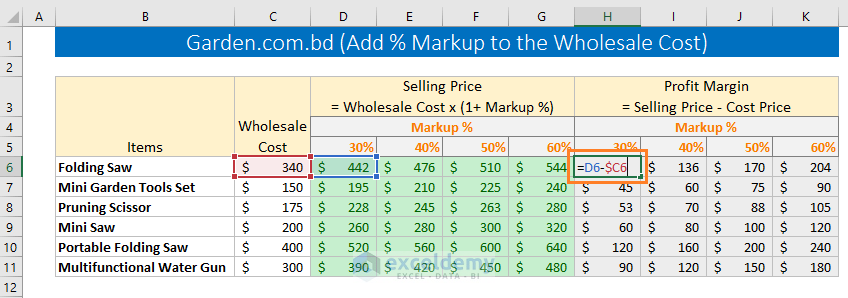## Calculating Selling Price, Cost Price, Markup % and Profit Margin % from One Another

1) Calculate Selling Price and Profit Margin % from the Cost Price and Markup %

In this calculation, Cost Price and Markup % are given, we calculate the Selling Price and the Profit Margin % from those data.

Here is the procedure: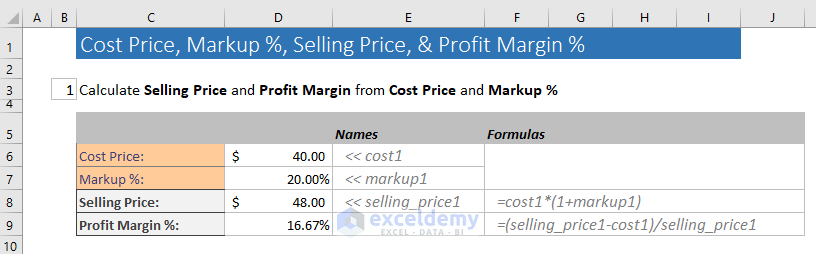2) Calculate Cost Price and Profit Margin % from Markup % and Selling Price.

In this calculation, Selling Price and Markup % are given. We calculate the Cost Price and Profit Margin % from them.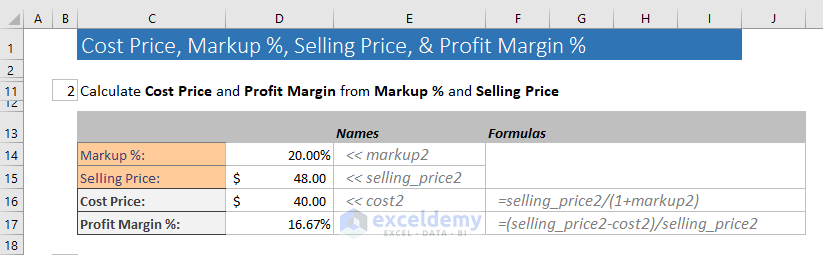3) Calculate Cost Price and Markup % from the Profit Margin % and the Selling Price.

In this calculation, Profit Margin % and Selling Price are given. We will calculate the Cost Price and Markup % from them.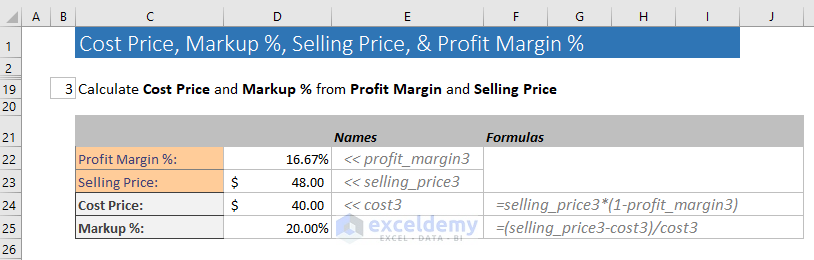## Markup % vs. Gross Profit Margin %

From our above discussion, it is clear now the difference between the Markup % and Gross Profit Margin %.

Using Markup %, we determine the Selling Price of a product based on the Cost Price.

We get Profit Margin % dividing the Profit Margin by the Selling Price.

Markup % varies from industry to industry.

 Industry Markup % Clothing 100-350% Shoes 100-500% Cell Phone 8-10% Furniture 200-400% Grocery 5-25% Cosmetics 60-80% Prescription Medicine 200-5600% New Car 8-10% Eyeglasses 800-1000% Source: Wise Bread

## Conclusion

Checking out your industry Markup % and determining the Selling Price of your product is important for becoming successful in your business. Being in the Shoe industry and accepting the Markup % of Grocery Industry will lead you to financial disaster.

So, these are my Excel formulas to add percentage markup to the cost price to get the selling price of a product. If you have any comments, please let me know in the comment box.

Thanks for being with my blog.

Happy Excelling!

Hello!
Welcome to my Excel blog! It took me some time to be a fan of Excel. But now I am a die-hard fan of MS Excel. I learn new ways of doing things with Excel and share here. Not only how to guide on Excel, but you will get also topics on Finance, Statistics, Data Analysis, and BI. Stay tuned!

1 Comment
1. Replythais Jan 3, 2020 at 4:04 PM

Hello, thank you fr the post, really helpful insights, but what if your product cost varies, for instance when importing, is there any way to create a formula to determine the margin when there is an increase or decrease? I’m struggling to get that one right